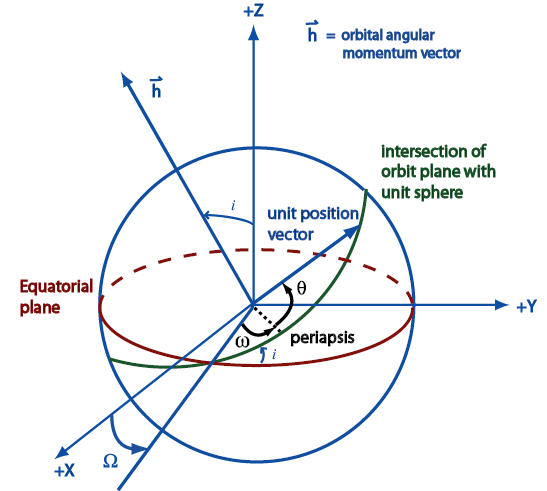Gets the inclination of the orbit, in radians.

Namespace:  AGI.Foundation.Coordinates
Assembly:  AGI.Foundation.OrbitPropagation (in AGI.Foundation.OrbitPropagation.dll) Version: 23.2.417.0 (23.2.417.0)Syntax
`public double Inclination { get; }`

#### Property Value

Type: DoubleRemarks

The inclination of the orbit is its tilt with reference to the Equatorial plane, or, in the diagram below, the angle i between the central body's spin (Z) axis and the orbit's angular momentum vector.In the above diagram, ω is the argument of periapsis, θ is the true anomaly, and Ω is the right ascension of the ascending node (RAAN).See Also